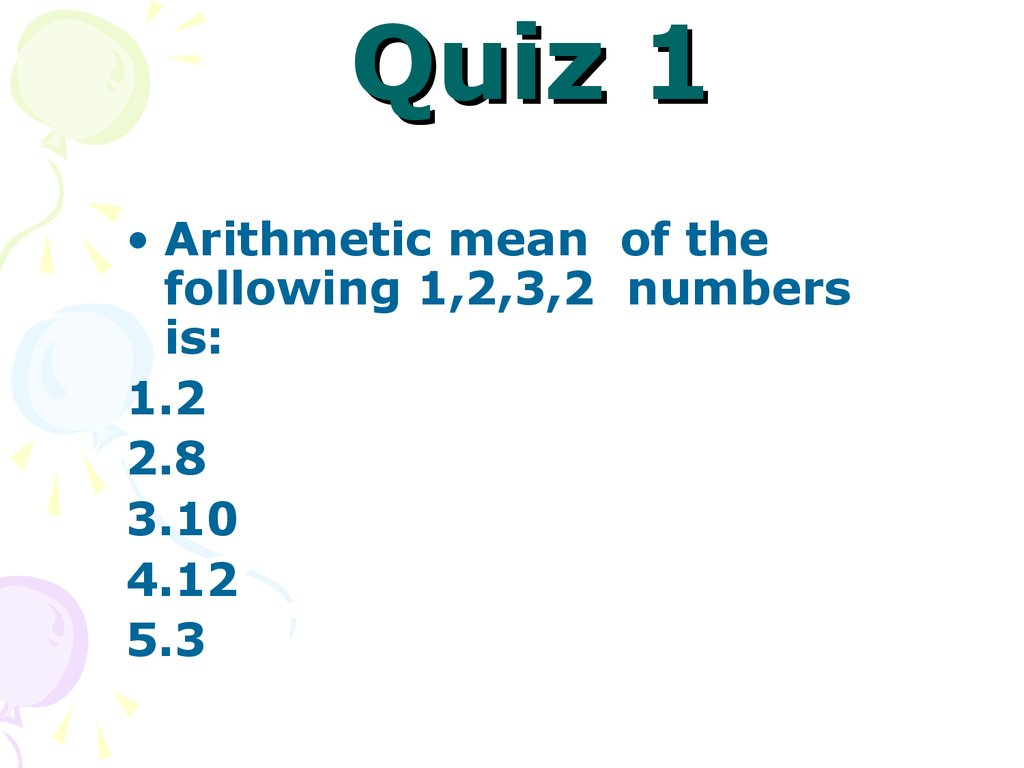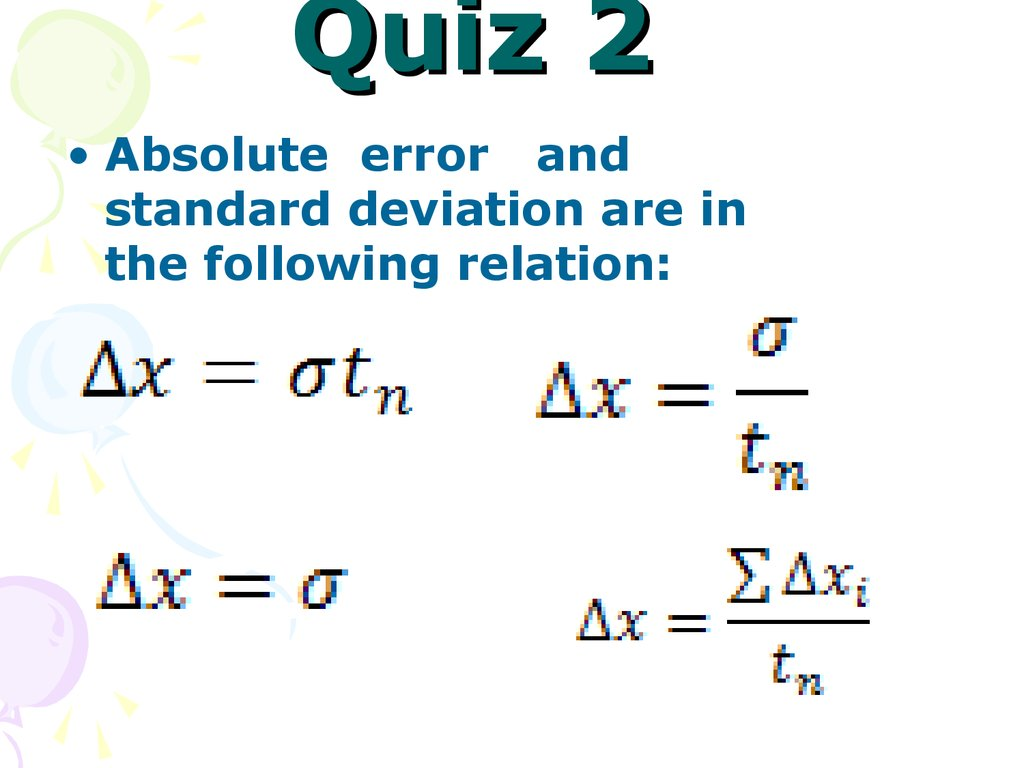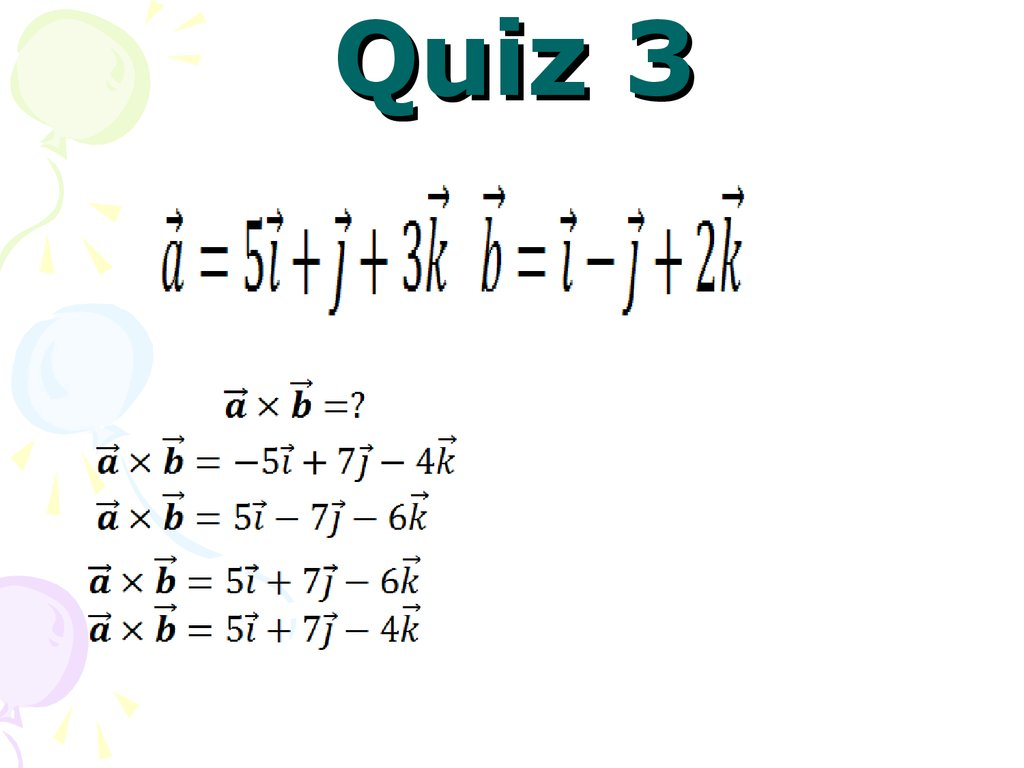# Quiz 1

## 1.

Quiz 1
• Arithmetic mean of the
following 1,2,3,2 numbers
is:
1.2
2.8
3.10
4.12
5.3

## 2.

Quiz 2
• Absolute error and
standard deviation are in
the following relation:

Quiz 3

## 4. Course of lectures «Contemporary Physics: Part1»

Part1
Lecture №2
Motion in One Dimension.
Motion in Two Dimensions.

## 5.

Position, Velocity, and Speed
Kinematics is the part of classical
mechanics,
which
describes
motion in terms of space and time
while ignoring the agents that
caused that motion.
The particle model — we describe
the moving object as a particle
regardless of its size. A particle is a
point-like object — that is, an object
with mass but having infinitesimal
size.

## 6.

Position, Velocity, and Speed
The displacement of a particle is
defined as its change in position in
some
time
interval.
The
displacement is a vector quantity
(1.1)
Distance is the length of a path
followed by a particle.
(1.2)
The average velocity
of a particle is
defined as the particle’s displacement Δx
divided by the time interval Δt during
which that displacement occurs.
The average speed of a particle, a scalar quantity, is
defined as the total distance traveled divided by the total time
interval required to travel that distance:
(1.3)

## 7.

Instantaneous Velocity and
Speed
The instantaneous speed of a
particle
is
defined
as
the
magnitude of its instantaneous
velocity.
(1.4)
(1.5)

## 8.

Acceleration
(1.6)
(1.7)
The
instantaneous
acceleration
equals the derivative of the velocity
with respect to time.
(1.8)

Acceleration

## 10.

Motion Diagrams
a) Motion diagram for a car moving at constant velocity (zero acceleration).
b) Motion diagram for a car whose constant acceleration is in the direction
of its velocity. The velocity vector at each instant is indicated by a red
arrow, and the constant acceleration by a violet arrow. c) Motion diagram
for a car whose constant acceleration is in the direction opposite the
velocity at each instant.

## 11.

One-Dimensional Motion
with Constant Acceleration

## 12.

One-Dimensional Motion
with Constant Acceleration
(2.9)
(2.10)
(2.11)
(2.9) → (2.11)
(2.12)
(2.13)

## 13.

One-Dimensional Motion
with Constant Acceleration

## 14.

(2.14)
is a displacement
vector

## 15.

The average velocity v of an object moving
through a displacement during a time interval
(Δt) is described by the formula
(2.15)
Note that the
average velocity
between points is
independent of
the path taken.

(2.16)

## 18.

Average acceleration

(2.17)

(2.18)

## 21.

Two-Dimensional Motion
with Constant Acceleration
If the position vector is known,
(2.19)
(2.20)

## 22.

Two-Dimensional Motion
with Constant Acceleration
(2.21)

## 23.

Two-Dimensional Motion
with Constant Acceleration
(2.22)

## 24.

Projectile Motion
(1) g is constant
over the range of
motion
and
is
directed Downward
(2) the effect of air
resistance
is
negligible

## 25.

Projectile Motion

## 26.

Projectile Motion
The vector expression for the position vector of the projectile as
a function of time
When analyzing projectile motion, consider
it to be the superposition of two motions:
(1)constant-velocity motion in the horizontal
direction and
(2)free-fall motion in the vertical direction.

## 27.

Even though an object moves at a constant
speed in a circular path, it still has an
acceleration.
Figure 2.5 (a) A car moving along a circular path at constant speed experiences
uniform circular motion. (b) As a particle moves from A to B, its velocity vector
changes from vi to vf . (c) The construction for determining the direction of the change
in velocity ∆v, which is toward the center of the circle for small ∆ r.

## 28.

The acceleration vector in uniform circular motion is always
perpendicular to the path and always points toward the center
of the circle. An acceleration of this nature is called a
centripetal acceleration (centripetal means center-seeking),
and its magnitude is
(2.23)
where r is the radius of the circle. The subscript on the
acceleration symbol reminds us that the acceleration is
centripetal.
For uniform circular motion, the acceleration vector can
only have a component perpendicular to the path, which is
toward the center of the circle.

## 29.

In many situations it is convenient to describe the motion
of a particle moving with constant speed in a circle of
radius r in terms of the period T, which is defined as the
time required for one complete revolution. In the time
interval T the particle moves a distance of 2πr, which is
equal to the circumference of the particle’s circular path.
Therefore, because its speed is equal to the circumference
of the circular path divided by the period, or v=2πr/T, it
follows that
(2.24)

## 30.

Figure 2.6. The motion of a particle along an arbitrary curved path lying
in the xy plane. If the velocity vector v (always tangent to the path)
changes in direction and magnitude, the components of the acceleration a
are a tangential component at and a radial component ar .

## 31.

The total acceleration vector a can be written as the vector sum of the
component vectors:
(2.25)
The tangential acceleration component causes the change in the
speed of the particle. This component is parallel to the instantaneous
velocity, and is given by
(2.26)
The radial acceleration component arises from the change in
direction of the velocity vector and is given by
(2.27)
where r is the radius of curvature of the path at the point in question.

## 32.

Quick Quiz 1 If a car is traveling eastward and
slowing down, what is the direction of the force on
the car that causes it to slow down? (a) eastward
(b) westward (c) neither of these.
Quick Quiz 2 A ball is thrown upward. While the
ball is in free fall, does its acceleration (a) increase
(b) decrease (c) increase and then decrease (d)
decrease and then increase (e) remain constant?
Quick Quiz 3 After a ball is thrown upward and is in
the air, its speed (a) increases (b) decreases (c)
increases and then decreases (d) decreases and
then increases (e) remains the same.